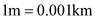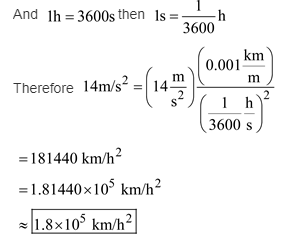# A Porsche sports car can accelerate at 14m/s2, (a) Is this acceleration greater than, less than, or equal

A Porsche sports car can accelerate at 14m/s2, (a) Is this acceleration greater than, less than, or equal to 14 ft/s2? Explain, (b) Determine the acceleration of a Forsche in ft/s2.
( c) Determine its acceleration in km/h2.

(a) The acceleration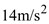is greater than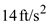(b) We have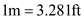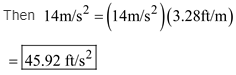( c) We have